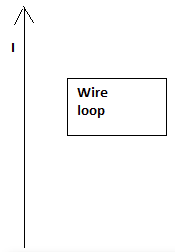# Problem: In the sketch to the right, a long straight wire is in the plane of a rectangular conducting wire loop. The current in the straight wire is up. At some point, the current in the straight wire starts to increase in magnitude. This increased current leads to an induced current in the wire loop. a) In which direction will the induced current in the wire loop be? Explain how you reached this conclusion. b) How would your answer be different if the wire loop was on the left side of the wire instead of the right side?

###### FREE Expert Solution

a)

Magnetic field is induced in the rectangular loop due to current in the wire.

As the current in the wire increases, the magnetic flux in the rectangular loop also increases.

An emf and current are induced according to Faraday's law.###### Problem Details

In the sketch to the right, a long straight wire is in the plane of a rectangular conducting wire loop. The current in the straight wire is up. At some point, the current in the straight wire starts to increase in magnitude. This increased current leads to an induced current in the wire loop.

a) In which direction will the induced current in the wire loop be? Explain how you reached this conclusion.

b) How would your answer be different if the wire loop was on the left side of the wire instead of the right side?